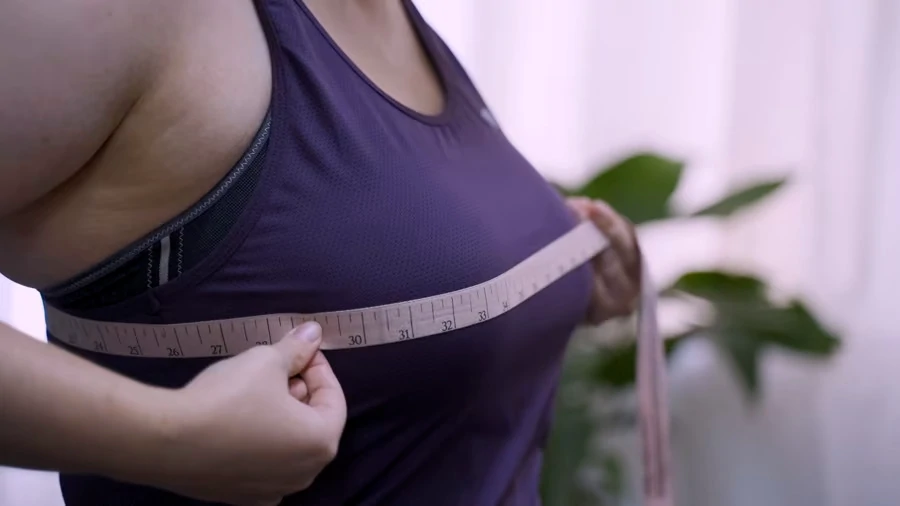🖤 FREE SHIPPING Australia wide with code SHIPITFREE 🖤

## How to Measure your Bra Size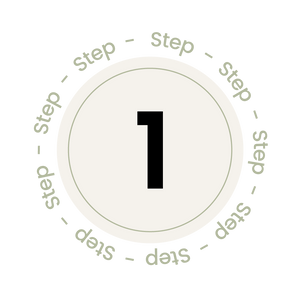Step One

Two measurements need to be taken. The rib cage circumference (measurement A) and the bust circumference (measurement B). Both measurements need to be recorded in inches.

The measuring tape should sit firmly, but not tightly against your skin. You should be breathing out when taking the measurements. When measuring, we recommend wearing your best fitted everyday bra.

Step Two

Measurement A will determine the band size.

28” = size 6                             38" = size 16

30” = size 8                             40" = size 18

32” = size 10                           42" = size 20

34” = size 12                           44" = size 22

36” = size 14                           46" = size 24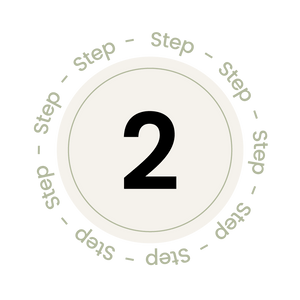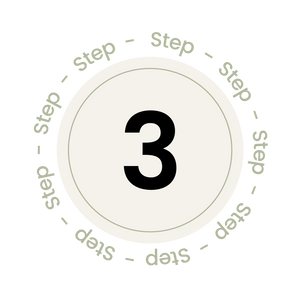Step Three

Determine your cup size by doing a simple calculation to measure the difference between the ribcage size and the bust size.

Measurement B (in inches) – Measurement A (in inches) = a difference of ??

Eg Measurement B = 40", Measurement A = 36” = difference of 4” = D cup

Eg Measurement B = 50”, Measurement A = 42” = difference of 8” = FF or G cup

Difference of 1 = A cup             Difference of 7 = F cup

Difference of 2 = B cup             Difference of 8 = FF/G cup

Difference of 3 = C cup             Difference of 9 = G/GG cup

Difference of 4 = D cup             Difference of 10 = GG/H cup

Difference of 5 = DD cup          Difference of 11 = H/HH cup

Difference of 6 = E cup             Difference of 12 = J cup

Step Four

## Put It All Together

If Measurement A is 32”, then the ribcage size should be a 10.

If Measurement B is 36”, then the difference between Measurement A and B is 4 inches, which will mean a cup size D. This example is a 10D.

Another example. If Measurement A is 38”, then the ribcage size should be a 16. If Measurement B is 45”, then the difference between Measurement A and B is 7 inches, which will mean a cup size F. This example is a 16F.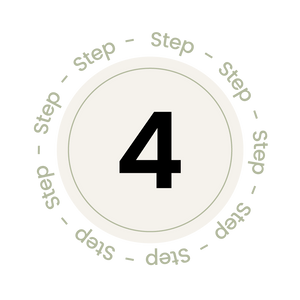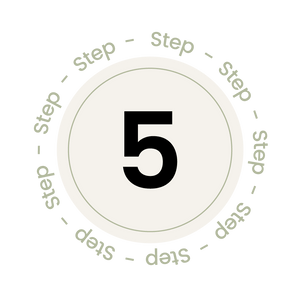Step Five

## Time to Troubleshoot!

You may not notice some inaccuracies in your measurements. But don't worry, this is not uncommon. The tape measurement system generally works well as a good guide.

If your size is not aligning with your expectations, reach out to us with some details about your measurements - we'd love to help!

#### Watch our tutorial video on how to measure your bra size